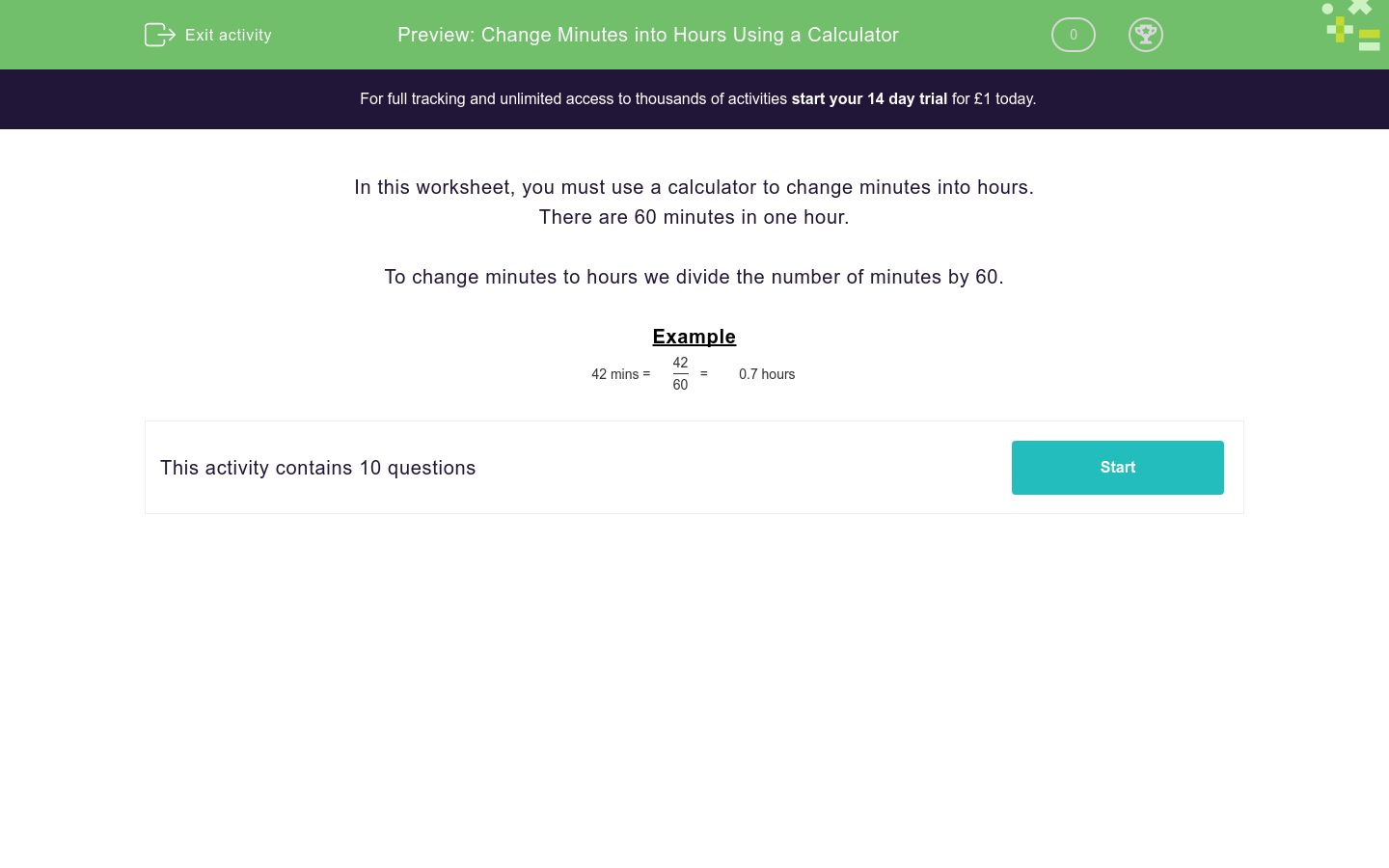# Change Minutes into Hours Using a Calculator

In this worksheet, students use a calculator to change minutes into hours.Key stage:  KS 3

Curriculum topic:   Number

Curriculum subtopic:   Use Calculators/Technology for Accuracy

Difficulty level:### QUESTION 1 of 10

In this worksheet, you must use a calculator to change minutes into hours.

There are 60 minutes in one hour.

To change minutes to hours we divide the number of minutes by 60.

Example

42 mins =
 42 60
=   0.7 hours

Change the following minutes to hours by dividing by 60.

6 mins  =
 ?
hrs.

(Just write the number in the text box below)

Change the following minutes to hours by dividing by 60.

3 mins  =
 ?
hrs.

(Just write the number in the text box below)

Change the following minutes to hours by dividing by 60.

39 mins  =
 ?
hrs.

(Just write the number in the text box below)

Change the following minutes to hours by dividing by 60.

9 mins  =
 ?
hrs.

(Just write the number in the text box below)

Change the following minutes to hours.

30 mins  =
 ?
hrs.

(Just write the number in the text box below)

Change the following minutes to hours.

33 mins  =
 ?
hrs.

(Just write the number in the text box below)

Change the following minutes to hours.

48 mins  =
 ?
hrs.

(Just write the number in the text box below)

Change the following minutes to hours.

42 mins  =
 ?
hrs.

(Just write the number in the text box below)

Change the following minutes to hours.

24 mins  =
 ?
hrs.

(Just write the number in the text box below)

Change the following minutes to hours.

51 mins  =
 ?
hrs.

(Just write the number in the text box below)

• Question 1

Change the following minutes to hours by dividing by 60.

6 mins  =
 ?
hrs.

(Just write the number in the text box below)

0.1
• Question 2

Change the following minutes to hours by dividing by 60.

3 mins  =
 ?
hrs.

(Just write the number in the text box below)

0.05
• Question 3

Change the following minutes to hours by dividing by 60.

39 mins  =
 ?
hrs.

(Just write the number in the text box below)

0.65
• Question 4

Change the following minutes to hours by dividing by 60.

9 mins  =
 ?
hrs.

(Just write the number in the text box below)

0.15
• Question 5

Change the following minutes to hours.

30 mins  =
 ?
hrs.

(Just write the number in the text box below)

0.5
• Question 6

Change the following minutes to hours.

33 mins  =
 ?
hrs.

(Just write the number in the text box below)

0.55
• Question 7

Change the following minutes to hours.

48 mins  =
 ?
hrs.

(Just write the number in the text box below)

0.8
• Question 8

Change the following minutes to hours.

42 mins  =
 ?
hrs.

(Just write the number in the text box below)

0.7
• Question 9

Change the following minutes to hours.

24 mins  =
 ?
hrs.

(Just write the number in the text box below)

0.4
• Question 10

Change the following minutes to hours.

51 mins  =
 ?
hrs.

(Just write the number in the text box below)

0.85
---- OR ----

Sign up for a £1 trial so you can track and measure your child's progress on this activity.

### What is EdPlace?

We're your National Curriculum aligned online education content provider helping each child succeed in English, maths and science from year 1 to GCSE. With an EdPlace account you’ll be able to track and measure progress, helping each child achieve their best. We build confidence and attainment by personalising each child’s learning at a level that suits them.

Get started